ISSN: 2322-0066

All submissions of the EM system will be redirected to Online Manuscript Submission System. Authors are requested to submit articles directly to Online Manuscript Submission System of respective journal.

# The Sextic Equation, its Algebraic Solution by Conversion to Solvable Factorized Form

Department of Mathematics/Physics Ngao Girls Secondary School, Kenya

*Corresponding Author:
Samuel BB
Department of Mathematics
Physics Ngao Girls Secondary School
Kenya
E-mail: [email protected]

Received date: 07/08/2017; Accepted date: 16/09/2017; Published date: 30/09/2017

Visit for more related articles at Research & Reviews: Research Journal of Biology

## Abstract

I present a method of solving the sextic equation by converting it to a solvable factorized form. The factorized form will consist of two cubic and their five parameters. The factorized form is selected such that four of its parameters are functionally related to the two parameters of the reduced sextic equation. The fifth parameter will be a rational number

#### Keywords

Sextic equation; Algebra; Septic equations

#### Introduction

Sextic equations are part of polynomial equations. Polynomial equations have many real world applications. The aim of this contribution is to further contribute to understanding of methods of solving sextic equations.

From literature polynomial equations were first investigated more than four thousand years ago[1,2].

The general sextic equation takes the form(1)

Abel and Ruffini showed that proved that it impossible to solve over a field of rational numbers.

Motlotle, in his 2011 master’s thesis managed to present a formula for solving the Bring-Jerrard quintic equation using the Newton’s sum formula. In his contribution Motlotle convincingly argued that Abel’s impossibility proof has been misconstrued by many as meaning that no general algebraic solution of the quintic equation is attainable. He showed that such a formula in unattainable only within a field of Rational numbers. He then moved on to deriving a formula. The importance this contribution is that formulae of higher degree polynomials are attainable at least over a field of algebraic numbers. Algebraic numbers include at least both radical and rational numbers. Algebraic numbers are solutions to polynomials with rational coefficients. The number field is constituted by both transcendental and algebraic numbers.

Thabo’s contribution is that for higher degree polynomial to have general solvable Galois group then the integral domain F (containing the parameters) should also contain the roots in the extension field. Dummit also contributed to sextic equations in his proof theorem.

In his theorem, he asserted that the irreducible quantic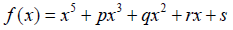is solvable in radicals if and only if the sextic equation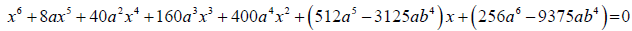has a rational root. If this is the case, the sextic factors into the product of a linear polynomial (x - θ) and an irreducible quintic, g(x).

I present a method of solving the sextic equation: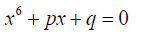(2)

In this contribution I present a solvable factorized form to which the above sextic equation can be converted to make it solvable. I will factor the above sextic into solvable quadratic and cubic factors.

The factorized form will carefully be selected so that their parameters can be correlated to those of the above quintic equation. If the parameters of the two auxiliary cubic factors are a, b, d, e and f and those of the sextic equation are p and q, the sextic equation has general algebraic solution if four of the parameters of the cubic and quadratic factors are functionally related to the two parameters of the sextic equation and the fifth parameter is a rational number.

Consider the sextic equation: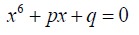(3)

Factorized form selected:(4)

The form selected above is similar to that used by  in his attempt to present a method to solve the general quintic equation.

If in the expansion of 4:

The x5 coefficient equated to zero then

d =1 (5)

The x4 coefficient is equated to zero and substituting 5 into the resulting equation:

b = 2a +1 (6)

The x3 coefficient is equated to zero and substituting 5 then we obtain the equation:

q + f ( f − e − a2 +1) = 0 (7)

Equating the x2 coefficient to zero and simplifying:

q + f (e − f ) = 0 (8)

Equating the x coefficient to p and simplifying:

eq + f2 = pf (9)

From 8(10)

Substituting 10 into 9 and simplifying:

f3 + f2 (q − p) − q2 = 0 (11)

Taking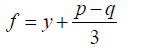(12)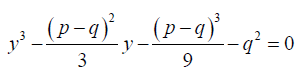(13)(14)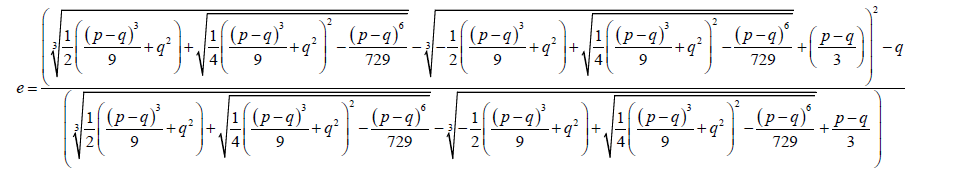(15)

From 15:(16)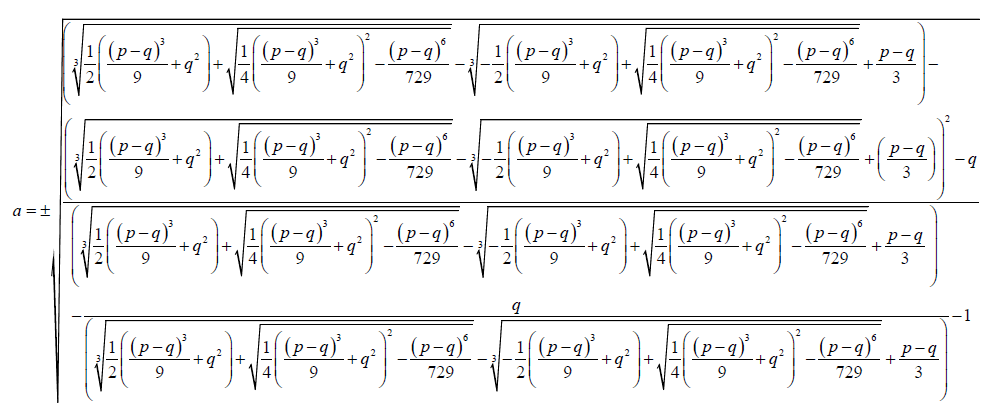(17)(18)

paper.io

agar io

wormax io